### GROWTH Function – Examples in Excel, VBA, Google Sheets

This Tutorial demonstrates how to use the Excel GROWTH Function in Excel to return values based on exponential growth.

## GROWTH Function Overview

The GROWTH Function Calculates Y values based on exponential growth rate for given X values. The exponential growth rate is calculated using two data series.

To use the GROWTH Excel Worksheet Function, select a cell and type: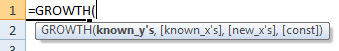(Notice how the formula inputs appear)

## GROWTH Function Syntax and inputs:

AutoMacro - VBA Code Generator

### Function Arguments ( Inputs ):

known_y’s – An array of known Y values.

known_x’s – An array of known X values.

new_x’s – An array of new X values that you want TREND to return corresponding Y values for.

const – OPTIONAL. Logical value indicating whether to calculate B (the intercept in y = mx + b) using the least squares method (TRUE Or Ommitted) or to manually set B = 0 (FALSE).

## What is GROWTH?

The GROWTH function in Excel is like the TREND function, used to predict an x value given known x and y values, except that it applies to exponential data. The GROWTH function uses the equation: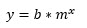where,

b =  y-intercept of the curve, and

m = the slope parameter of the curve, which can also be written as .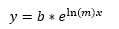Using this alternate form of m, the equation can also be written as .

## How to use GROWTH

The GROWTH function takes four arguments:

Where, known_y’s and known_x’s refer to the x and y data in your data table, new_x’s is an array of data points you wish to predict the y-value for and const is a binary argument for calculating the y-intercept normally, or forcing the value to 1 and adjusting the slope values so that .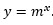Although the latter 3 arguments of GROWTH are optional, it is recommended to include all arguments to ensure the data prediction is correct.

Let us look at an example: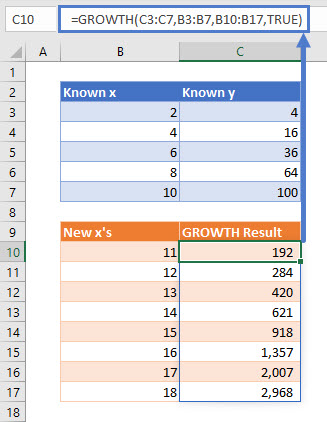Using GROWTH in Microsoft 365 (namely, the Office Insider Current Channel (Preview)), and all array functions, act as a dynamic array formula which spills the result for the entire array, as shown with the light blue border around cells C10 to C17 in this example. Simply type the formula in cell C10 and hit enter, no need to fill or drag the formula down.

To show the exponential equation that GROWTH found to predict our results, I have plotted the data and used Excel’s built in trendline equation: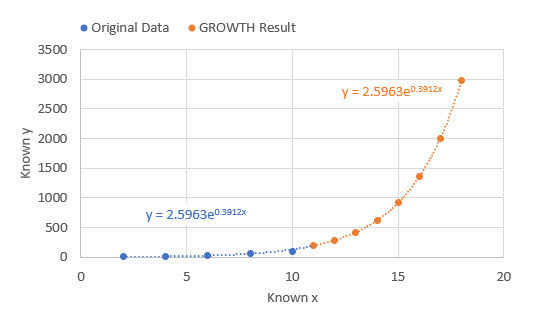The equations match!

# GROWTH TIPS

1. Ensure you have the most updated version of Microsoft 365 to utilize GROWTH with dynamic arrays. You may need to enable the Office Insider Current Channel (Preview) to utilize dynamic array functions. On the Account Page: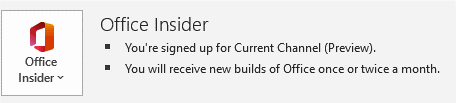(Not required to use the function, just for dynamic array functionality)

1. If your data is formatted as an Excel table (usually by Ctrl+T), GROWTH will result in errors. Spilled array formulas are not supported inside of Excel tables. Move your data out of the table and try again.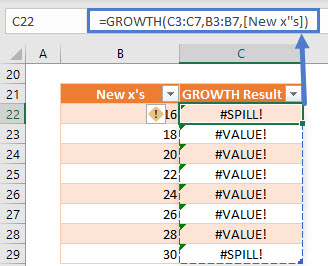1. If your new x’s array is not full, you will get a #VALUE! Error. Change the range in the function argument so it is continuous.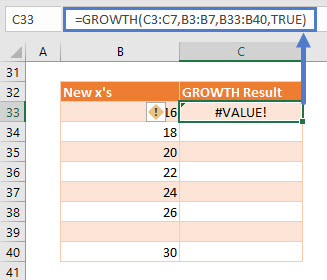## Interested in More Forecasting?

See our other articles on Forecasting with Exponential Smoothing, TREND, LINEST and LOGEST functions.

## GROWTH function in Google Sheets

The GROWTH function works exactly the same in Google Sheets as in Excel.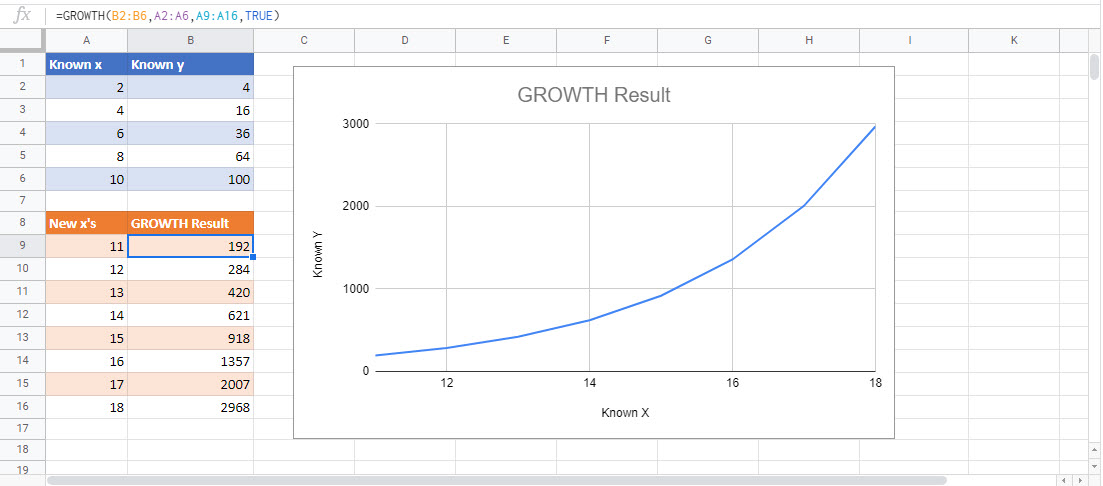## GROWTH Examples in VBA

You can also use the GROWTH function in VBA. Type:
`application.worksheetfunction.growth(known_ys,known_x,new_x,const)`
For the function arguments (known_y’s, etc.), you can either enter them directly into the function, or define variables to use instead.

Return to the List of all Functions in Excel

## Excel Practice Worksheet

Practice Excel functions and formulas with our 100% free practice worksheets!

• Automatically Graded Exercises
• Learn Excel, Inside Excel!